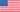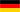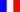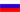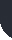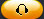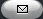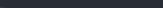Task Management Software for Team Collaboration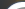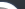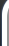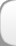HomeDownloadSupportPurchase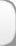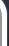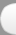Installation GuideStartUp GuideOnline Help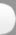# Profit Rate per Each Unit Sold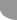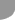Home  » Products  » VIP Task Manager Pro  » Tutorials  » Customization Guide  » Profit Rate per Each Unit Sold

Profit Rate per Each Unit Sold

Profit Rate per Each Unit Sold refers to [Gross Profit Margin]. Profit Rate shows how much profit you get from each dollar of the product/service cost per some time span. The profit excludes any costs associated with the production of a product or service: the cost of raw materials, labor costs, transportation costs, etc. Profit Rate per Each Unit Sold is calculated by the formula:

Profit Rate per Each Unit Sold = Profit per unit / Revenue per unit

Profit per unit= Revenue per unit - Cost of production per unit

Revenue per Unit = Cost you sell your product or service.

To calculate Cost of production per unit the following formula is used:

Cost of production per unit = Cost of Goods Sold (COGS) / Number of units produced within some period of time.

Cost of Goods Sold (COGS) = Cost of raw materials + labor cost + transportation cost + other costs associated with the production. Actually the formula to calculate COGS differs from company to company.

Alternatively you can use the formula for calculating COGS by the Weighted Average Method.

Profit Rate per Each Unit Sold is one more measure that allows to estimate the companys progress.

To calculate Profit rate per Each Unit Sold please follow the steps below:

• Create the custom field "COGS" (Cost of Goods Sold) with Code "cf_cogs"
• Add the cost of raw materials + labor cost + transportation cost + other costs that refer to production process.
• To calculate COGS automatically you can use the following formula:

\$cf_raw_materials_cost + \$cf_labor_cost + \$cf_transportation_cost + \$cf_other_costs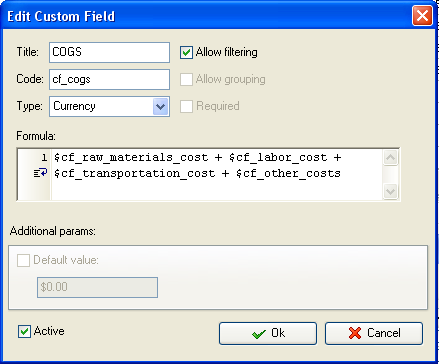If you use the Weighted Average Method to calculate COGS the formula may look like this:

\$cf_quantity_purchases * \$cf_average_cost_per_unit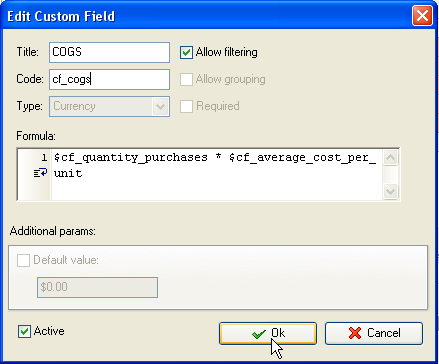As the value of the custom field "COGS" is the same for all units you sell, you can enter it in "Default value" field after calculating COGS for the whole project: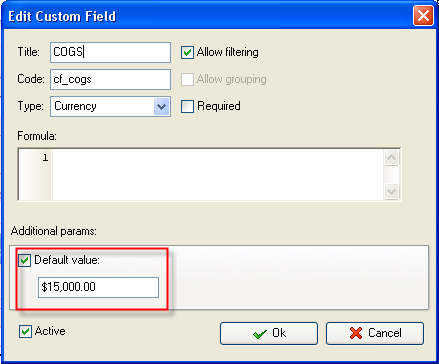• Create the custom field "Cost of production per unit" with Code "cf_cost_of_production_per_unit" and the formula:
• \$cf_cogs / X, where X is the total number of units produced over some period of time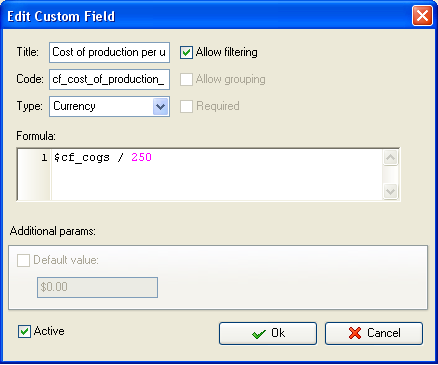• Create the custom field "Revenue per unit" with Code "cf_revenue_per_unit". Add the cost you sell your product.
•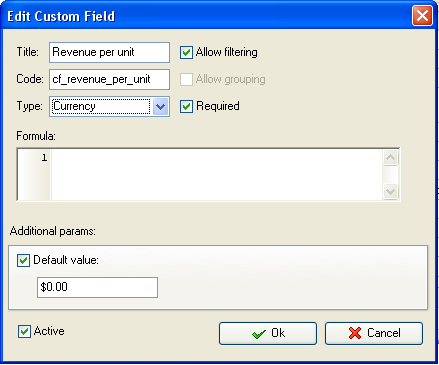• Add the custom field "Profit per unit" with Code "cf_profit_per_unit" the formula:
•  \$cf_revenue_per_unit - \$cf_cost_of_production_per_unit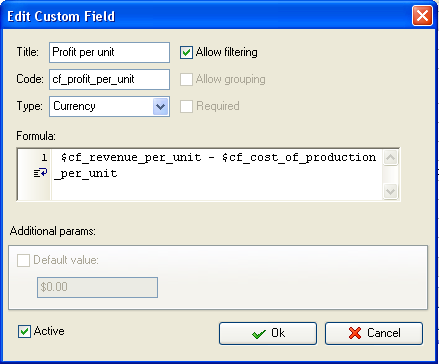• Create the custom field "Profit Rate per Each Unit Sold" with the formula:
• \$cf_profit_per_unit / \$cf_revenue_per_unit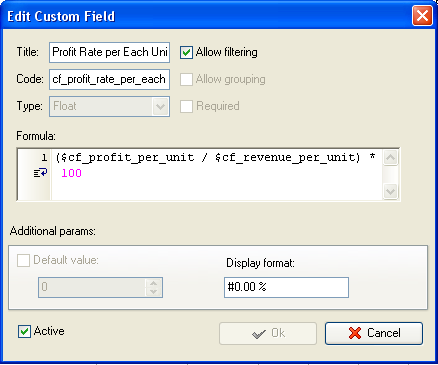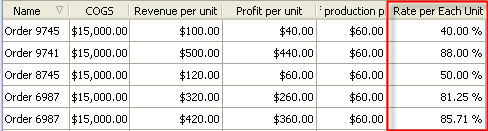Profit Rate per Each Unit Sold on Task List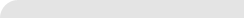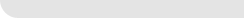» VIP Task Manager Pro VIP Task Manager Std Testimonials Turnkey Solution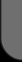ProductsSolutionsDownloadSupportPurchaseContactsForumVideoMy Account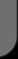Copyright © 2004 - 2023 Task Management Software